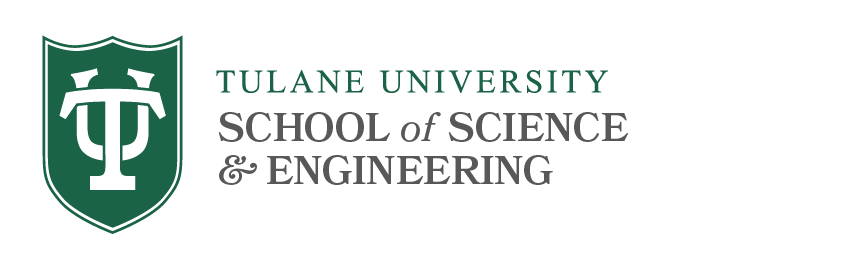# 2000 Level Courses

Math 2170 Discrete Mathematics (3)
Prerequisite: Math 1220 or 1310.
An introduction to the concepts and techniques of discrete Mathematics including set theory, Mathematical induction, graphs, trees, ordered sets, Boolean algebras, and the basic laws of combinatorics.

Math 2210 Calculus III (4)
Prerequisite: Math 1220 or 1310.
A basic course in differential and integral calculus of several variables. Vectors in the plane and space. Vector functions, derivatives, arc length, curvature. Functions of several variables: continuity, partial derivatives, chain rule, gradient, optimization, Lagrange multipliers. Double and triple integrals: change of variables, polar coordinates, cylindrical and spherical coordinates, surface area. Vector fields: gradient, curl, divergence, line and surface integrals, Green’s, Stokes’, and Divergence theorems.

Math 2240 Introduction to Applied Mathematics (4)
Prerequisite: Math 1220 or 1310.
An introduction to the techniques of applied Mathematics. The emphasis will be on the Mathematical modeling by differential equations of a variety of applications in the natural sciences. Numerical and graphical techniques for finding both quantitative and qualitative information about solutions will be discussed and implemented on the computer. No programming experience is assumed. Students may not receive credit for both 2240 and 4240.